• Harris角点检测器 定义 角点: 像素点周围显示存在多余一个方向的边，也称为兴趣点 数学解释 把图像域中点 xxx 上的对称半定矩阵 MI=MI(x)M_I = M_I(x)MI​=MI​(x) 定义为 MI=▽I▽IT=[IxIy][IxIy]=[Ix2IxIyIxIyIy...
Harris角点检测器
定义
角点:

像素点周围显示存在多余一个方向的边，也称为兴趣点

数学解释
把图像域中点 $x$ 上的对称半定矩阵 $M_I = M_I(x)$ 定义为
$M_I=▽I▽I^T= \begin{bmatrix}I_x\\I_y\end{bmatrix} \begin{bmatrix}I_x&I_y\end{bmatrix} = \begin{bmatrix}I_x^2&I_xI_y\\I_xI_y&I_y^2\end{bmatrix}$
$▽I$ 为包含导数 $I_xI_y$ 的图像梯度，即图像变化的强弱
根据该定义 $M_I$ 的秩为 $1$，特征值为 $λ_1=|▽I|^2$ 和 $λ_2=0$
通过选择权重矩阵 $W$ (gaussian_filter) 得到卷积 ($M_I$ 在周围像素上的局部平均)
$\overline{M_I} = W * M_I$
矩阵$\overline{M_I}$就是 Harris矩阵
$W$ 的宽度决定了像素 $x$ 周围的兴趣区域
$M_I$ 在周围像素上的局部平均，其特征值会依赖局部图像特性而变化，即梯度改变。
取决于该区域$▽I$的值，Harris矩阵 $\overline{M_I}$ 的特征值有三种情况

如果 $λ_1 λ_2$ 是很大的正数，则该 $x$ 为角点；
如果 $λ_1$ 很大，$λ_2≈0$，则该区域内存在一个边，该区域内的平均 $\overline{M_I}$ 的特征值不会变化太大；
如果 $λ_1≈λ_2≈0$，该区域为空。

代码
    def compute_harris_response(im,sigma=3):
"""在一副灰度图像中，对每个像素计算Harris角点检测器响应函数"""

#计算导数
imx = zeros(im.shape)
filters.gaussian_filter(im,(sigma,sigma),(0,1),imx)
imy = zeros(im.shape)
filters.gaussian_filter(im,(sigma,sigma),(1,0),imy)

#计算Harris矩阵的分量
Wxx = filters.gaussian_filter(imx * imx,sigma)
Wxy = filters.gaussian_filter(imx * imy,sigma)
Wyy = filters.gaussian_filter(imy * imy,sigma)

#计算特征值和迹
Wdet = Wxx * Wyy - Wxy ** 2
Wtr = Wxx + Wyy

return Wdet / Wtr

    def get_harris_points(harrisim,min_dist=10,threshold=0.05):
""" 从一副Haris响应图像中返回角点。 min_dist为分割角点和图像边界的最少像素数目"""

#寻找高于阈值的候角点
corner_threshold = harrisim.max() * threshold
harrisim_t = (harrisim > corner_threshold) * 1

#得到候选点的坐标
coords = array(harrisim_t.nonzero()).T

#以及它们的Harris响应值
candidate_values = [harrisim[c,c]for c in coords]

#对候选点按照Harris响应值进行排序
index = argsort(candidate_values)

#将可行点的位置保存到数组中
allowed_locations = zeros(harrisim.shape)
allowed_locations[min_dist:-min_dist,min_dist:-min_dist] = 1

#按照min_distance 原则 ，选择最佳Harris点
filtered_coords = []
for i in index:
if allowed_locations[coords[i,0],coords[i,0]] == 1:
filtered_coords.append(coords[i])
allowed_locations[(coords[i,0] - min_dist):(coords[i,0] + min_dist),
(coords[i,1] - min_dist):(coords[i,1] + min_dist)] = 0

return filtered_coords

    def plot_harris_points(image,filtered_coords):
"""绘制图像中检测到的角点"""

figure()
gray()
imshow(image)
plot([p for p in filtered_coords],[p for p in filtered_coords],'.')
axis('off')
show()

运行效果原图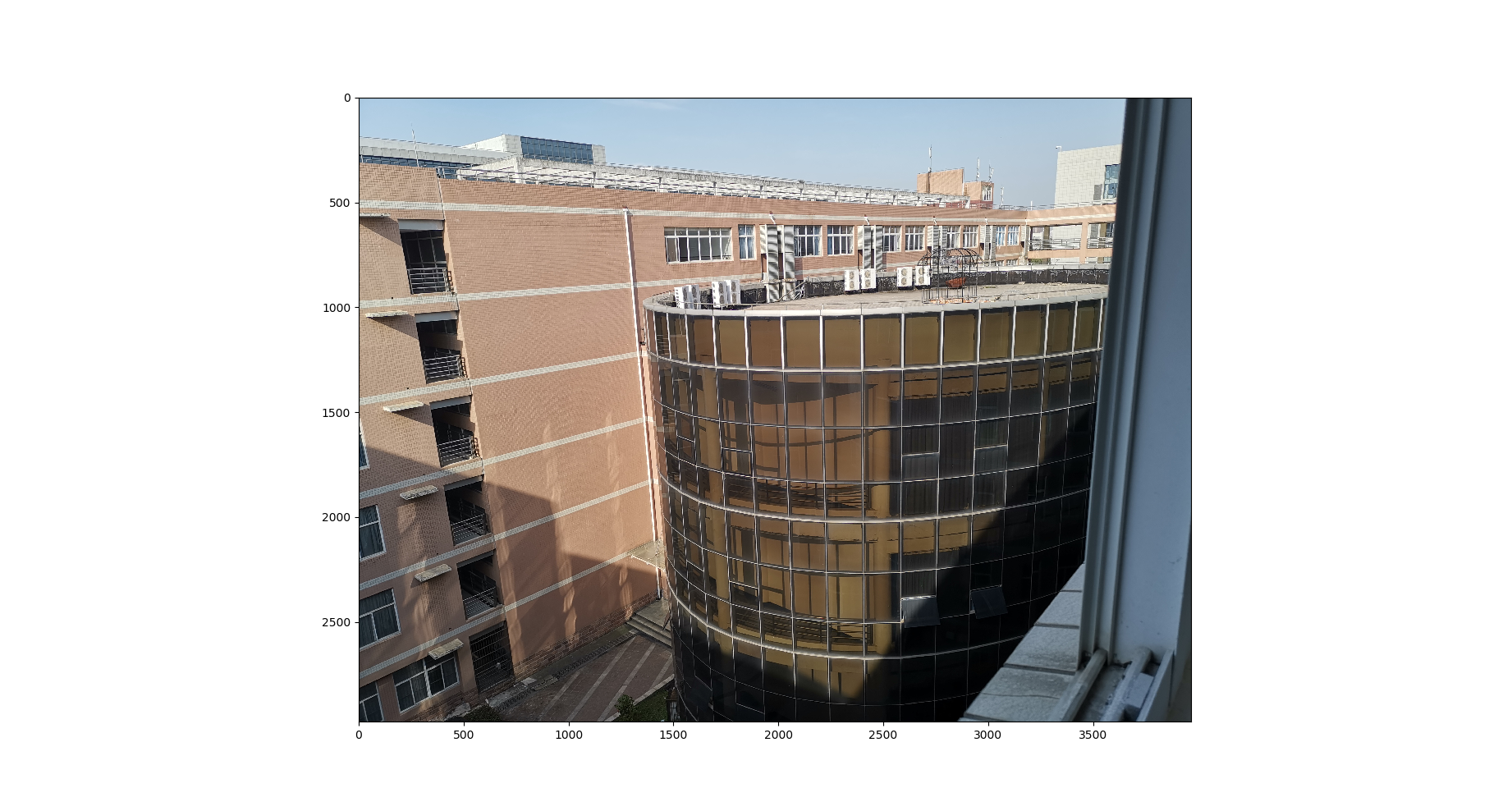序列化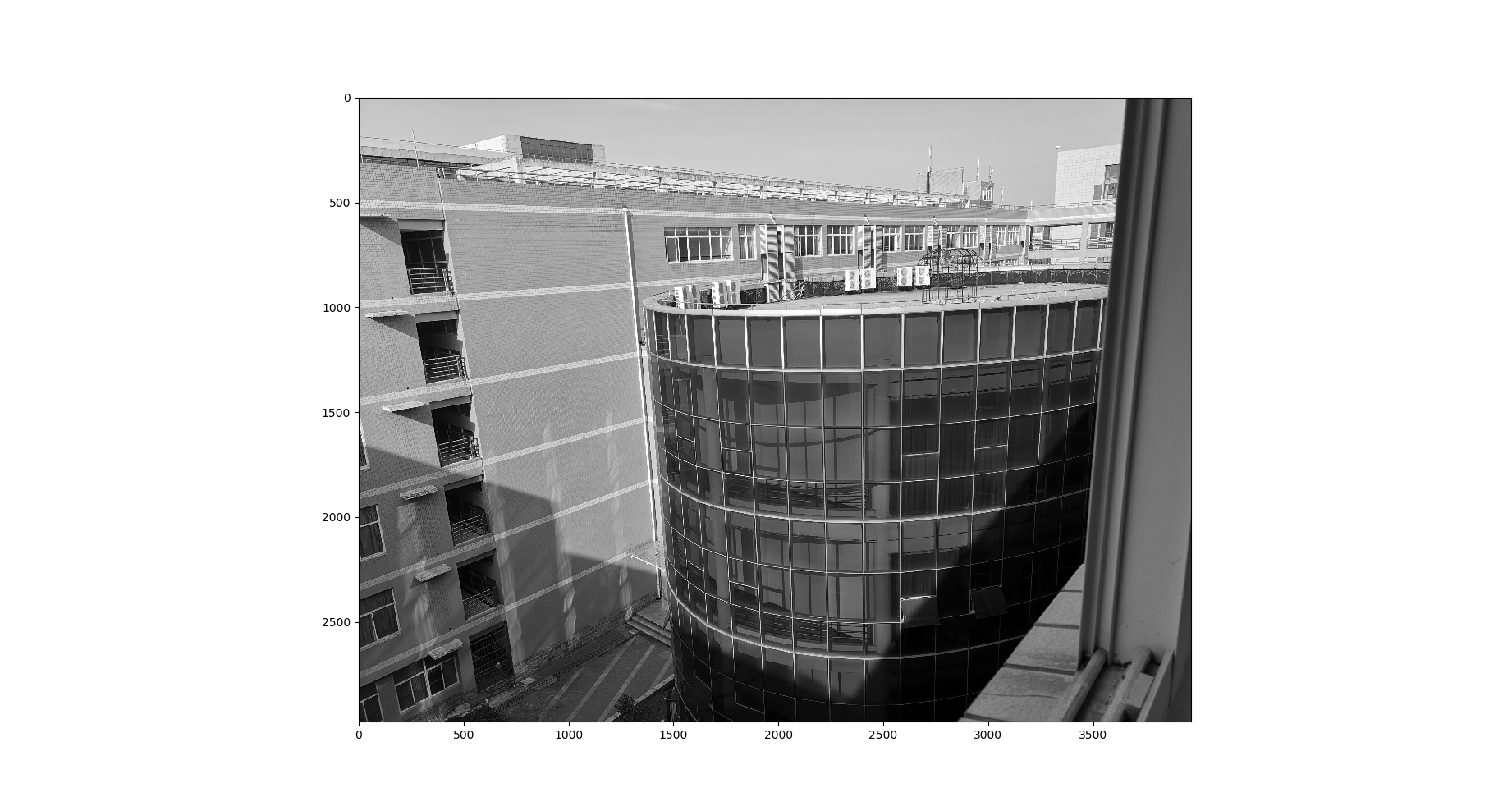灰度图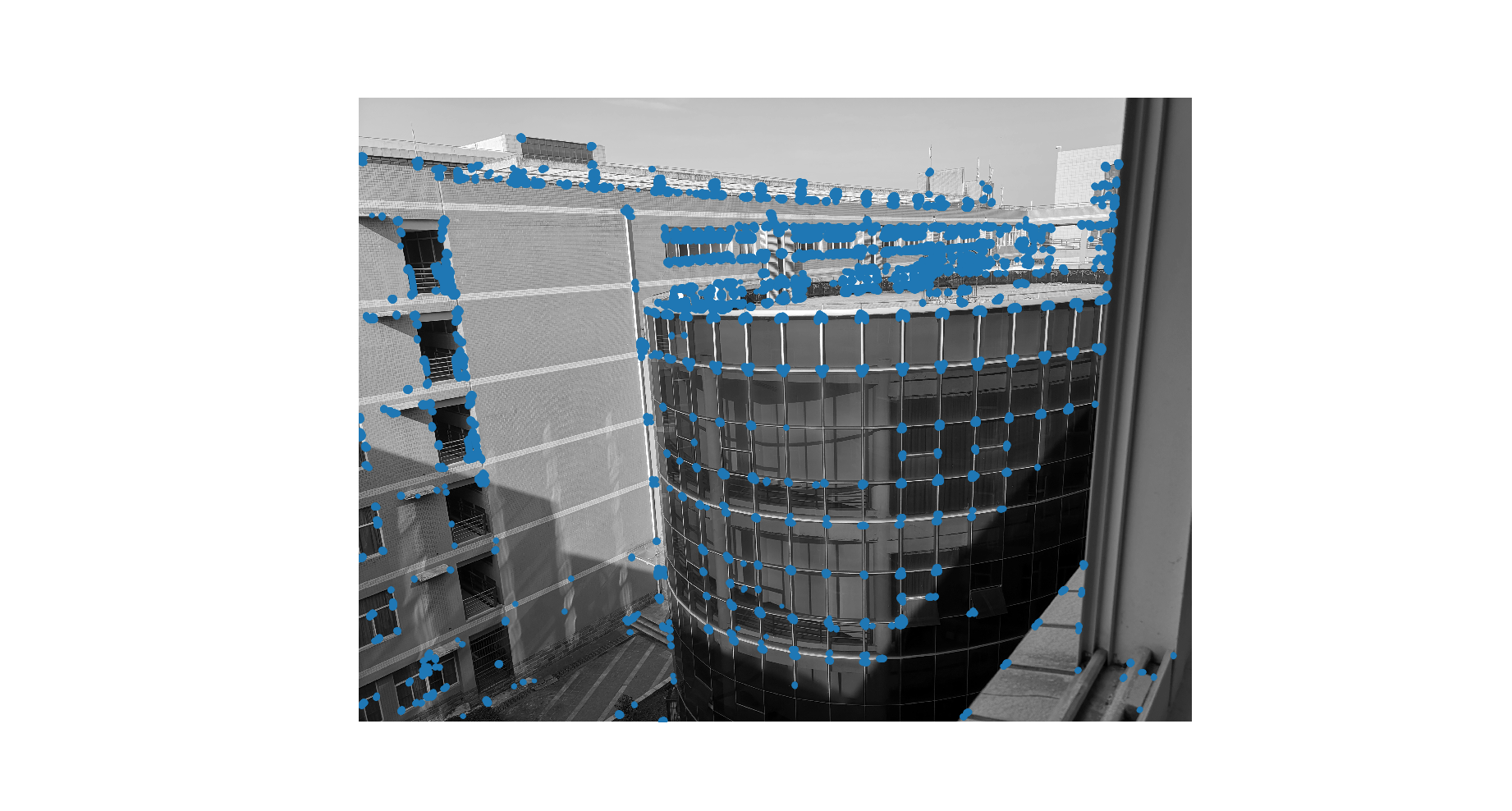阈值为0.1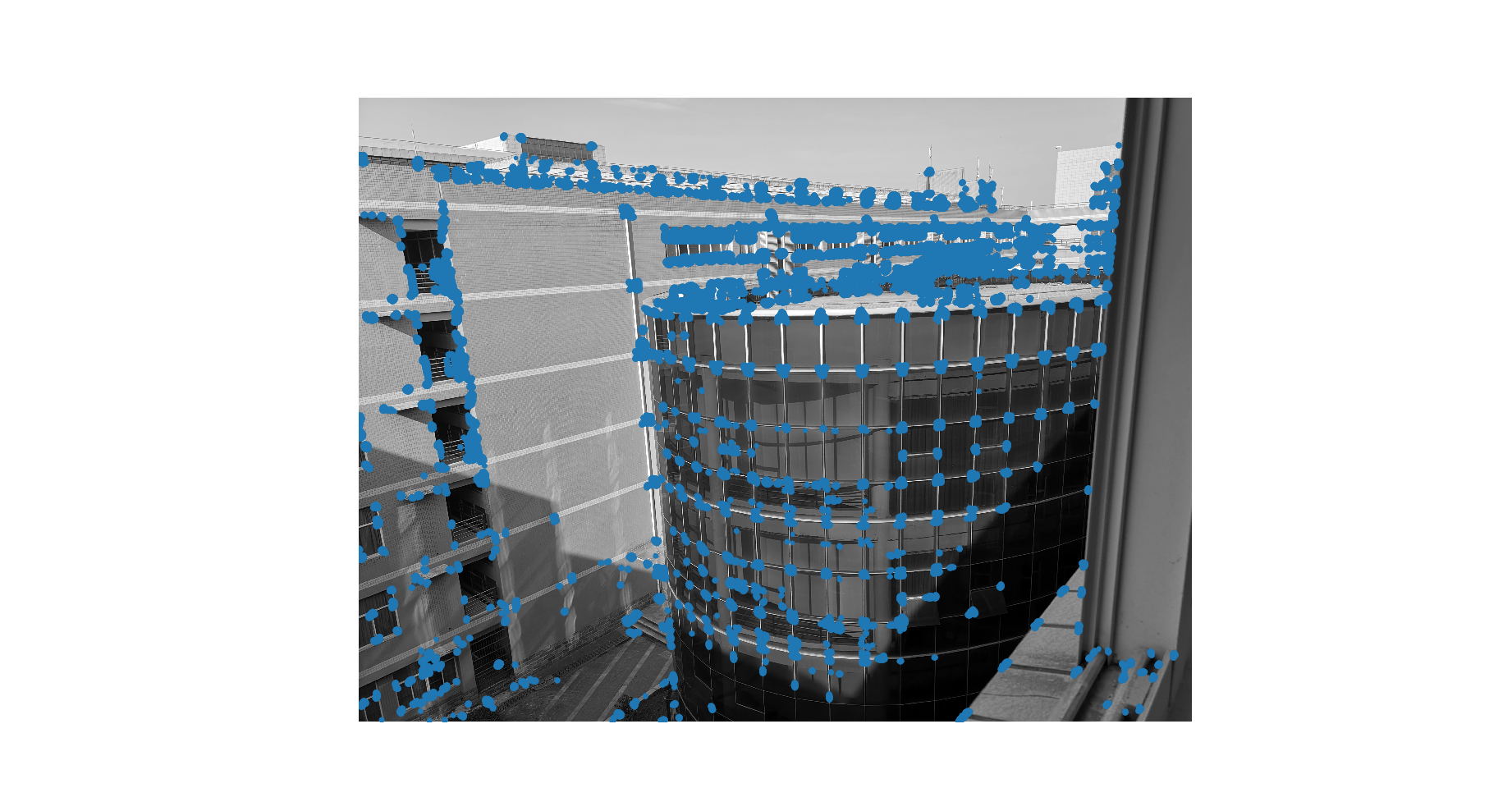阈值为0.05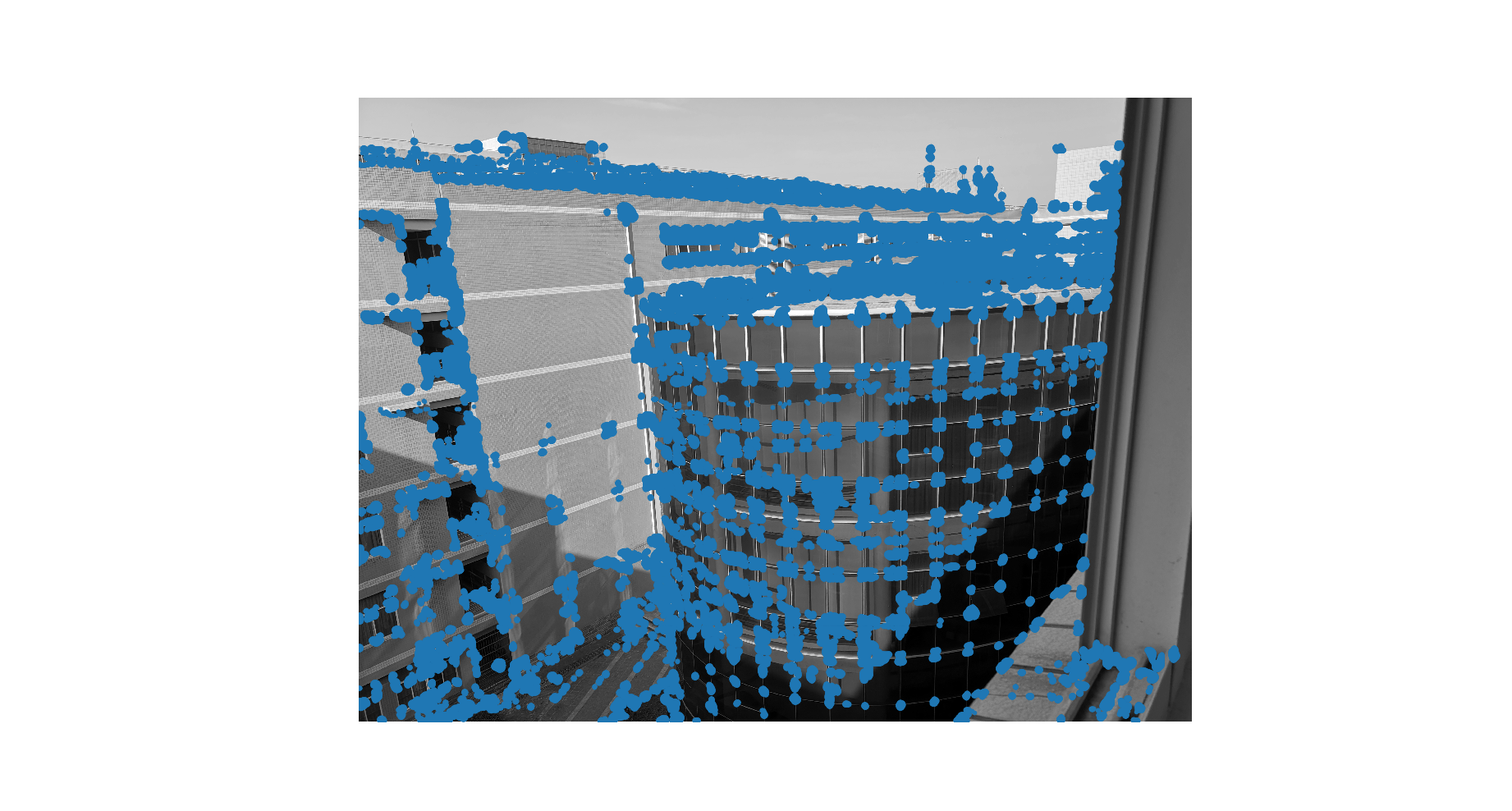阈值为0.01
总结
该方法为一种局部图像描述子算法，用于创建全景图、增强现实技术以及计算图像的三维重建。
这种算法是一种极为简单的角点检测算法，效果受限于图片，对一些图片可能存在无效等问题。


展开全文图像处理
• Harris角点检测器1.对图像进行高斯滤波。 2.对每个像素，估计其垂直方向的梯度大小值。使用近似于导数的核做两次一维卷积即可。 3.对每一像素核给定的邻域窗口： 计算局部结构矩阵A 计算响应函数R(A) 4....
Harris角点检测器1.对图像进行高斯滤波。
2.对每个像素，估计其垂直方向的梯度大小值。使用近似于导数的核做两次一维卷积即可。
3.对每一像素核给定的邻域窗口：
计算局部结构矩阵A
计算响应函数R(A)
4.选取响应函数R(A)的一个阈值，以选择最佳候选角点，并完成非最大化抑制。
Harris角点检测算子是于1988年由CHris Harris & Mike Stephens提出来的。在具体展开之前，不得不提一下Moravec早在1981就提出来的Moravec角点检测算子。
1.Moravec角点检测算子
Moravec角点检测算子的思想其实特别简单，在图像上取一个W*W的“滑动窗口”，不断的移动这个窗口并检测窗口中的像素变化情况E。像素变化情况E可简单分为以下三种：A  如果在窗口中的图像是什么平坦的，那么E的变化不大。B  如果在窗口中的图像是一条边，那么在沿这条边滑动时E变化不大，而在沿垂直于这条边的方向滑动窗口时，E的变化会很大。 C  如果在窗口中的图像是一个角点时，窗口沿任何方向移动E的值都会发生很大变化。上图就是对Moravec算子的形象描述。用数学语言来表示的话就是：其中（x，y）就表示四个移动方向（1，0）（1，1）（0，1）（-1，1），E就是像素的变化值。Moravec算子对四个方向进行加权求和来确定变化的大小，然和设定阈值，来确定到底是边还是角点。

2.Harris角点检测算子
Harris角点检测算子实质上就是对Moravec算子的改良和优化。在原文中，作者提出了三点Moravec算子的缺陷并且给出了改良方法：
1.  Moravec算子对方向的依赖性太强，在上文中我们可以看到，Moravec算子实际上只是移动了四个45度角的离散方向，真正优秀的检测算子应该能考虑到各个现象的移动变化情况。为此，作者采用微分的思想（这里不清楚的话可以复习下高数中的全微分）：其中：所以E就可以表示为：2.由于Moravec算子采用的是方形的windows，因此的E的响应比较容易受到干扰，Harris采用了一个较为平滑的窗口——高斯函数：3.Moravec算子对边缘响应过于灵敏。为此，Harris提出了对E进行变形：对，没错，变成了二次型，果然是大牛，更牛的还在后面！其中，用α，β表示矩阵M的特征值，这样会产生三种情况：A  如果α，β都很小，说明高斯windows中的图像接近平坦。 B  如果一个大一个小，则表示检测到边。 C  如果α，β都很大，那么表示检测到了角点。
α，β是什么？α，β就是椭圆的长短轴的度量，什么？椭圆哪里来？椭圆就是那个二次型函数来的！下图的意思我就不详细讲解了，相信大家能懂。
{{
转载注：NewThinker_wei：
关于矩阵知识的一点补充：好长时间没看过线性代数的话，这一段比较难理解。可以看到M是实对称矩阵，这里简单温习一下实对称矩阵和二次型的一些知识点吧。
1. 关于特征值和特征向量：
特征值的特征向量的概念忘了就自己查吧，这里只说关键的。对于实对称矩阵M（设阶数为n），则一定有n个实特征值，每个特征值对应一组特征向量（这组向量中所有向量共线），不同特征值对应的特征向量间相互正交；（注意这里说的是实对称矩阵，不是所有的矩阵都满足这些条件）
2. 关于对角化：
对角化是指存在一个正交矩阵Q，使得  Q’MQ 能成为一个对角阵（只有对角元素非0），其中Q’是Q的转置（同时也是Q的逆，因为正交矩阵的转置就是其逆）。一个矩阵对角化后得到新矩阵的行列式和矩阵的迹（对角元素之和）均与原矩阵相同。如果M是n阶实对称矩阵，则Q中的第 j 列就是第 j 个特征值对应的一个特征向量（不同列的特征向量两两正交）。
3. 关于二次型：
对于一个n元二次多项式，f(x1,x2....xn) = ∑ ( aij*xi*xj ) ，其中 i 和 j 的求和区间均为 [1,n] ，
可将其各次的系数 aij 写成一个n*n矩阵M，由于 aij 和 aji 的对称等价关系，一般将 aij 和 aji 设为一样的值，均为 xi*xj 的系数的二分之一。这样，矩阵M就是实对称矩阵了。即二次型的矩阵默认都是实对称矩阵
4. 关于二次型的标准化（正交变换法）：
二次型的标准化是指通过构造一个n阶可逆矩阵 C，使得向量 ( x1,x2...xn ) = C * (y1,y2...yn)，把n维向量 x 变换成n维向量 y ，并代入f(x1,x2....xn) 后得到 g(y1,y2...yn)，而后者的表达式中的二次项中不包含任何交叉二次项 yi*yj（全部都是平方项 yi^2），也即表达式g的二次型矩阵N是对角阵。用公式表示一下 f 和 g ，（下面的表达式中 x 和 y都代表向量，x' 和 y' 代表转置）
f = x' * M * x ;
g = f = x' * M * x = (Cy)' * M * (Cy) = y' * (C'MC) * y = y' * N * y  ;
因此 C‘MC = N。正交变换法，就是直接将M对角化得到N，而N中对角线的元素就是M的特征值。正交变换法中得到的 C 正好是一个正交矩阵，其每一列都是两两正交的单位向量，因此 C 的作用仅仅是将坐标轴旋转（不会有放缩）。

OK，基础知识补充完了，再来说说Harris角点检测中的特征值是怎么回事。这里的 M 是将M对角化后得到矩阵N，他们都是2阶矩阵，且N的对角线元素就是本文中提到的 α 和 β。
本来 E(x,y) = A*x^2 + 2*C*x*y + B*y^2 ，而将其标准后得到新的坐标 xp和yp，这时表达式中就不再含有交叉二次项，新表达式如下：
E(x,y) = Ep (xp,yp) = α*xp^2 + β*yp^2，
我们不妨画出 Ep (xp,yp) = 1 的等高线L ，即
α*xp^2 + β*yp^2 = 1 ，
可见这正好是(xp,yp)空间的一个椭圆，而α 和 β则分别是该椭圆长、短轴平方的倒数（或者反过来），且长短轴的方向也正好是α 和 β对应的特征向量的方向。由于(x,y)空间只是 (xp,yp)空间的旋转，没有放缩，因此等高线L在(x,y)空间也是一个全等的椭圆，只不过可能是倾斜的。
现在就能理解下面的图片中出现的几个椭圆是怎么回事了，图(a)中画的正是高度为 1 的等高线，（其他”高度“处的等高线也是椭圆，只不过长短轴的长度还要乘以一个系数）。其他的几幅图片中可以看到，“平坦”区域由于（高度）变化很慢，等高线（椭圆）就比较大；而”边缘“区域则是在一个轴向上高度变化很快，另一个与之垂直的轴向上高度变化很慢，因此一个轴很长一个轴很短；“角点”区域各个方向高度都变化剧烈，因此椭圆很小。我们人眼可以直观地看到椭圆的大小胖瘦，但如何让计算机识别这三种不同的几何特征呢？为了能区分出角点、边缘和平坦区域我们现在需要用α 和 β构造一个特征表达式，使得这个特征式在三种不同的区域有明显不同的值。一个表现还不错的特征表达式就是：
(αβ) - k(α+β)^2
表达式中的 k 的值怎么选取呢？它一般是一个远小于 1 的系数，opencv的默认推荐值是 0.04(=0.2的平方)，它近似地表达了一个阈值：当椭圆短、长轴的平方之比（亦即α 和 β两个特征值之比）小于这个阈值时，认为该椭圆属于“一个轴很长一个轴很短”，即对应的点会被认为是边缘区域。
对于边缘部分，（假设较大的特征值为β）由于 β>>α且α<kβ，因此特征式 ：
(αβ) - k(α+β)^2 ≈ αβ - kβ^2  <  (kβ)β - kβ^2 = 0
即边缘部分的特征值小于0 ；
对于非边缘部分，α 和 β相差不大，可认为 (α+β)^2 ≈ 4αβ，因此特征式：

(αβ) - k(α+β)^2 ≈ αβ - 4kαβ =  ( 1 - 4k ) * αβ
由于 k 远小于1，因此 1 - 4k ≈ 1，这样特征式进一步近似为：
(αβ) - k(α+β)^2 ≈  αβ

在角点区域，由于α 和 β都较大，对应的特征式的值也就很大；而在平坦区域，特征式的值则很小。

因此，三种不同区域的判别依据就是： 如果特征表达式的值为负，则属于边缘区域；如果特征表达式的值较大，则属于角点区域；如果特征表达式的值很小，则是平坦区域。
最后，由于αβ和(α+β)正好是M对角化后行列式和迹，再结合上面补充的基础知识第2条中提到的行列式和迹在对角化前后不变，就可以得到 (αβ) - k(α+β)^2 = det(M) - k*Tr(M)^2，这就是Harris检测的表达式。

转载注解完毕，下面接原文。
}}有人又要问了，你怎么知道我检测到α，β算大还是算小？对此天才哈里斯定义了一个角点响应函数：其中（这些都是线性代数里的知识）：我们惊喜的发现,R为正值是，检测到的是角点，R为负时检测到的是边，R很小时检测到的是平坦区域。至于他怎么想出来的，我就不得而知了......Harris角点检测算法有诸多优点：A  旋转不变性，椭圆转过一定角度但是其形状保持不变（特征值保持不变）B  对于图像灰度的仿射变化具有部分的不变性，由于仅仅使用了图像的一介导数，对于图像灰度平移变化不变；对于图像灰度尺度变化不变
当然Harris也有许多不完善的地方：A  它对尺度很敏感，不具备几何尺度不变性。B  提取的角点是像素级的。

close all;
clear all;
clc;

img=imread('rice.png');
imshow(img);
[m n]=size(img);

tmp=zeros(m+2,n+2);
tmp(2:m+1,2:n+1)=img;
Ix=zeros(m+2,n+2);
Iy=zeros(m+2,n+2);

E=zeros(m+2,n+2);

Ix(:,2:n)=tmp(:,3:n+1)-tmp(:,1:n-1);
Iy(2:m,:)=tmp(3:m+1,:)-tmp(1:m-1,:);

Ix2=Ix(2:m+1,2:n+1).^2;
Iy2=Iy(2:m+1,2:n+1).^2;
Ixy=Ix(2:m+1,2:n+1).*Iy(2:m+1,2:n+1);

h=fspecial('gaussian',[7 7],2);
Ix2=filter2(h,Ix2);
Iy2=filter2(h,Iy2);
Ixy=filter2(h,Ixy);

Rmax=0;
R=zeros(m,n);
for i=1:m
for j=1:n
M=[Ix2(i,j) Ixy(i,j);Ixy(i,j) Iy2(i,j)];
R(i,j)=det(M)-0.06*(trace(M))^2;

if R(i,j)>Rmax
Rmax=R(i,j);
end
end
end
re=zeros(m+2,n+2);

tmp(2:m+1,2:n+1)=R;
img_re=zeros(m+2,n+2);
img_re(2:m+1,2:n+1)=img;
for i=2:m+1
for j=2:n+1

if tmp(i,j)>0.01*Rmax &&...
tmp(i,j)>tmp(i-1,j-1) && tmp(i,j)>tmp(i-1,j) && tmp(i,j)>tmp(i-1,j+1) &&...
tmp(i,j)>tmp(i,j-1) && tmp(i,j)>tmp(i,j+1) &&...
tmp(i,j)>tmp(i+1,j-1) && tmp(i,j)>tmp(i+1,j) && tmp(i,j)>tmp(i+1,j+1)
img_re(i,j)=255;
end

end
end

figure,imshow(mat2gray(img_re(2:m+1,2:n+1)));转载于:https://www.cnblogs.com/hfutemg/p/5611329.html
展开全文人工智能
• Stephens 角点检测器）是一个极为简单的角点检测算法。该算法的主要思想是，如果像素周围显示存在多于一个方向的边，我们认为该点为兴趣点。该点就称为角点。from PIL import Image from numpy import * from pylab...
Harris 角点检测算法（也称Harris & Stephens 角点检测器）是一个极为简单的角点检测算法。该算法的主要思想是，如果像素周围显示存在多于一个方向的边，我们认为该点为兴趣点。该点就称为角点。from PIL import Image
from numpy import *
from pylab import *
from scipy.ndimage import filters

def compute_harris_response(im,sigma=3):
""" 在一幅灰度图像中，对每个像素计算Harris 角点检测器响应函数"""
# 计算导数
imx = zeros(im.shape)
filters.gaussian_filter(im, (sigma,sigma), (0,1), imx)
imy = zeros(im.shape)
filters.gaussian_filter(im, (sigma,sigma), (1,0), imy)
# 计算Harris 矩阵的分量
Wxx = filters.gaussian_filter(imx*imx,sigma)
Wxy = filters.gaussian_filter(imx*imy,sigma)
Wyy = filters.gaussian_filter(imy*imy,sigma)
# 计算特征值和迹
Wdet = Wxx*Wyy - Wxy**2
Wtr = Wxx + Wyy
return Wdet / Wtr

def get_harris_points(harrisim,min_dist=10,threshold=0.1):
""" 从一幅Harris 响应图像中返回角点。min_dist 为分割角点和图像边界的最少像素数目"""
# 寻找高于阈值的候选角点
corner_threshold = harrisim.max() * threshold
harrisim_t = (harrisim > corner_threshold) * 1
# 得到候选点的坐标
coords = array(harrisim_t.nonzero()).T
# 以及它们的Harris 响应值
candidate_values = [harrisim[c,c] for c in coords]
# 对候选点按照Harris 响应值进行排序
index = argsort(candidate_values)
# 将可行点的位置保存到数组中
allowed_locations = zeros(harrisim.shape)
allowed_locations[min_dist:-min_dist,min_dist:-min_dist] = 1
# 按照min_distance 原则，选择最佳Harris 点
filtered_coords = []
for i in index:
if allowed_locations[coords[i,0],coords[i,1]] == 1:
filtered_coords.append(coords[i])
allowed_locations[(coords[i,0]-min_dist):(coords[i,0]+min_dist),
(coords[i,1]-min_dist):(coords[i,1]+min_dist)] = 0
return filtered_coords

def plot_harris_points(image,filtered_coords):
""" 绘制图像中检测到的角点"""
figure()
gray()
imshow(image)
plot([p for p in filtered_coords],[p for p in filtered_coords],'*')
axis('off')
show()

im = array(Image.open('ji.jpg').convert('L'))
harrisim = compute_harris_response(im)
filtered_coords = get_harris_points(harrisim,6)
plot_harris_points(im, filtered_coords)   输出：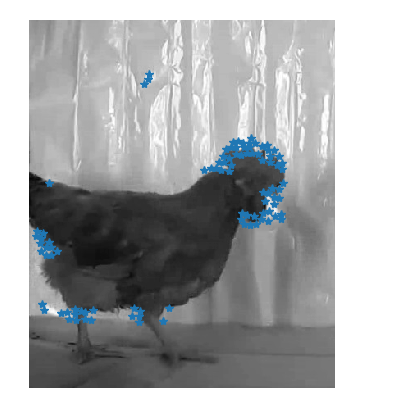Harris角点检测器检测图像中的兴趣点harris.pyfrom PIL import Image

from pylab import *
from scipy.ndimage import filters

def compute_harris_response(im,sigma=3):
""" 在一幅灰度图像中，对每个像素计算Harris 角点检测器响应函数"""
# 计算导数
imx = zeros(im.shape)
filters.gaussian_filter(im, (sigma,sigma), (0,1), imx)
imy = zeros(im.shape)
filters.gaussian_filter(im, (sigma,sigma), (1,0), imy)
# 计算Harris 矩阵的分量
Wxx = filters.gaussian_filter(imx*imx,sigma)
Wxy = filters.gaussian_filter(imx*imy,sigma)
Wyy = filters.gaussian_filter(imy*imy,sigma)
# 计算特征值和迹
Wdet = Wxx*Wyy - Wxy**2
Wtr = Wxx + Wyy
return Wdet / Wtr

def get_harris_points(harrisim,min_dist=10,threshold=0.1):
""" 从一幅Harris 响应图像中返回角点。min_dist 为分割角点和图像边界的最少像素数目"""
# 寻找高于阈值的候选角点
corner_threshold = harrisim.max() * threshold
harrisim_t = (harrisim > corner_threshold) * 1
# 得到候选点的坐标
coords = array(harrisim_t.nonzero()).T
# 以及它们的Harris 响应值
candidate_values = [harrisim[c,c] for c in coords]
# 对候选点按照Harris 响应值进行排序
index = argsort(candidate_values)
# 将可行点的位置保存到数组中
allowed_locations = zeros(harrisim.shape)
allowed_locations[min_dist:-min_dist,min_dist:-min_dist] = 1
# 按照min_distance 原则，选择最佳Harris 点
filtered_coords = []
for i in index:
if allowed_locations[coords[i,0],coords[i,1]] == 1:
filtered_coords.append(coords[i])
allowed_locations[(coords[i,0]-min_dist):(coords[i,0]+min_dist),
(coords[i,1]-min_dist):(coords[i,1]+min_dist)] = 0
return filtered_coords

def plot_harris_points(image,filtered_coords):
""" 绘制图像中检测到的角点"""
figure()
gray()
imshow(image)
plot([p for p in filtered_coords],[p for p in filtered_coords],'*')
axis('off')
show()

def get_descriptors(image,filtered_coords,wid=5):
""" 对于每个返回的点，返回点周围2*wid+1 个像素的值（假设选取点的min_distance > wid）"""
desc = []
for coords in filtered_coords:
patch = image[coords-wid:coords+wid+1,
coords-wid:coords+wid+1].flatten()
desc.append(patch)
return desc

def match(desc1,desc2,threshold=0.5):
""" 对于第一幅图像中的每个角点描述子，使用归一化互相关，选取它在第二幅图像中的匹配角点"""
n = len(desc1)
# 点对的距离
d = -ones((len(desc1),len(desc2)))
for i in range(len(desc1)):
for j in range(len(desc2)):
d1 = (desc1[i] - mean(desc1[i])) / std(desc1[i])
d2 = (desc2[j] - mean(desc2[j])) / std(desc2[j])
ncc_value = sum(d1 * d2) / (n-1)
if ncc_value > threshold:
d[i,j] = ncc_value
ndx = argsort(-d)
matchscores = ndx[:,0]
return matchscores

def match_twosided(desc1,desc2,threshold=0.5):
""" 两边对称版本的match()"""
matches_12 = match(desc1,desc2,threshold)
matches_21 = match(desc2,desc1,threshold)
ndx_12 = where(matches_12 >= 0)
# 去除非对称的匹配
for n in ndx_12:
if matches_21[matches_12[n]] != n:
matches_12[n] = -1
return matches_12

def appendimages(im1,im2):
""" 返回将两幅图像并排拼接成的一幅新图像"""
# 选取具有最少行数的图像，然后填充足够的空行
rows1 = im1.shape
rows2 = im2.shape
if rows1 < rows2:
im1 = concatenate((im1,zeros((rows2-rows1,im1.shape))),axis=0)
elif rows1 > rows2:
im2 = concatenate((im2,zeros((rows1-rows2,im2.shape))),axis=0)
# 如果这些情况都没有，那么它们的行数相同，不需要进行填充
return concatenate((im1,im2), axis=1)

def plot_matches(im1,im2,locs1,locs2,matchscores,show_below=True):
""" 显示一幅带有连接匹配之间连线的图片
输入：im1，im2（数组图像），locs1，locs2（特征位置），matchscores（match() 的输出），
show_below（如果图像应该显示在匹配的下方）"""
im3 = appendimages(im1,im2)
if show_below:
im3 = vstack((im3,im3))
imshow(im3)
cols1 = im1.shape
for i,m in enumerate(matchscores):
if m>0:
plot([locs1[i],locs2[m]+cols1],[locs1[i],locs2[m]],'c')
axis('off')
main.pyimport harris
from numpy import *
from pylab import *
from PIL import Image

im1 = array(Image.open('hcl.jpg').convert('L'))
im2 = array(Image.open('ln.jpg').convert('L'))

wid = 5

harrisim = harris.compute_harris_response(im1,5)
filtered_coords1 = harris.get_harris_points(harrisim,wid+1)
d1 = harris.get_descriptors(im1,filtered_coords1,wid)

harrisim = harris.compute_harris_response(im2,5)
filtered_coords2 = harris.get_harris_points(harrisim,wid+1)
d2 = harris.get_descriptors(im2,filtered_coords2,wid)

print ('starting matching')
matches = harris.match_twosided(d1,d2)
figure()
gray()
harris.plot_matches(im1,im2,filtered_coords1,filtered_coords2,matches)
show()
展开全文•matlab
• 第二章讲局部图像描述子，旨在寻找图像间的对应点和对应区域。可以通过图像匹配的方式完成创建全景图、增强现实技术以及计算图像的三维重建等工作。...# 2.1 Harris角点检测器 from pylab import * from numpy ...
第二章讲局部图像描述子，旨在寻找图像间的对应点和对应区域。可以通过图像匹配的方式完成创建全景图、增强现实技术以及计算图像的三维重建等工作。

Harris角点检测算法（也称Harris或Stephens角点检测器）），主要思想：如果像素周围显示存在多于一个方向的边，就认为该点为兴趣点。称为角点。

# 2.1 Harris角点检测器
from pylab import *
from numpy import *
from PIL import Image
from numpy import  random
from scipy.ndimage import filters
from imageio import imwrite

def computer_harris_response(im, sigma = 3):
"""在一幅灰度图像中，对每个像素计算Harris角点检测器响应函数"""

# 计算导数
imx = zeros(im.shape)
filters.gaussian_filter(im, (sigma, sigma), (0, 1), imx)
imy = zeros(im.shape)
filters.gaussian_filter(im, (sigma, sigma), (1, 0), imy)
# 计算Harris矩阵的分量
Wxx = filters.gaussian_filter(imx*imx, sigma)
Wxy = filters.gaussian_filter(imx * imy, sigma)
Wyy = filters.gaussian_filter(imy * imy, sigma)
# 计算特征值和迹
Wdet = Wxx*Wyy-Wxy**2
Wtr = Wxx + Wyy

return Wdet/Wtr

def get_harris_points(harrisim, min_dist=10, threshold=0.1):
"""从一幅Harris响应图像中返回角点。min_dist为分割角点和图像边界的最小像素数目"""

# 寻找高于阈值的候选角点
corner_threshold = harrisim.max()*threshold
harrisim_t = (harrisim > corner_threshold)*1
# 得到候选点的坐标
coords = array(harrisim_t.nonzero()).T
# 以及它们的Harris响应值
candidate_values = [harrisim[c, c] for c in coords]
# 对候选点按照Harris响应值进行排序
index = argsort(candidate_values)
# 将可行点的位置保存到数组中
allowed_locations = zeros(harrisim.shape)
allowed_locations[min_dist:-min_dist, min_dist:-min_dist] = 1
# 按照min_distance原则，选择最佳Harris点
filtered_coords = []
for i in index:
if allowed_locations[coords[i,0], coords[i,1]] == 1:
filtered_coords.append(coords[i])
allowed_locations[(coords[i,0]-min_dist):(coords[i,0]+min_dist),
(coords[i,1]-min_dist):(coords[i,1]+min_dist)] = 0

return filtered_coords

def plot_harris_points(image, filtered_coords):
"""绘制图像中检测到的角点"""

figure()
gray()
imshow(image)
plot([p for p in filtered_coords], [p for p in filtered_coords], '*')
axis('off')
show()

im = array(Image.open('empire.jpg').convert('L'))
harrisim = computer_harris_response(im)
filtered_coords = get_harris_points(harrisim, threshold=0.2)
plot_harris_points(im, filtered_coords)

以上为一个Harris角点检测算法实现的例子。如果想要了解角点检测的不同方法，包括Harris角点检测器的改进和进一步的开发应用，可以查找资源，如下面：

网站

在图像间寻找对应点

from pylab import *
from numpy import *
from PIL import Image
from numpy import random
from scipy.ndimage import filters
from imageio import imwrite

def get_descriptors(image, filtered_coords, wid=5):
"""
对于每个返回的点，返回点周围2*wid+1个像素的值
（假设选取点的min_distance>wid)
"""
desc = []
for coords in filtered_coords:
patch = image[coords - wid:coords + wid + 1,
coords - wid:coords + wid + 1].flatten()
desc.append(patch)
return desc

def match(desc1, desc2, threshold=0.5):
"""对于第一幅图像中的每个角点描述子，使用归一化互相关，
选取它在第二幅图像中的匹配角点"""
n = len(desc1)
# 点对的距离
d = -ones((len(desc1), len(desc2)))
for i in range(len(desc1)):
for j in range(len(desc2)):
d1 = (desc1[i]-mean(desc1[i]))/std(desc1[i])
d2 = (desc2[j]-mean(desc2[j]))/std(desc2[j])
ncc_value = sum(d1*d2)/(n-1)  # 归一化的互相关矩阵
if ncc_value > threshold:
d[i, j] = ncc_value

ndx = argsort(-d)  # Returns the indices that would sort an array. 从小到大
matchscores = ndx[:, 0]

return matchscores

def match_twosided(desc1, desc2, threshold=0.5):
"""两边对称版本的match()"""
matches_12 = match(desc1, desc2, threshold)
matches_21 = match(desc2, desc1, threshold)

ndx_12 = where(matches_12 >= 0)

# 去除非对称的匹配
for n in ndx_12:
if matches_21[matches_12[n]] != n:
matches_12[n] = -1

return matches_12

def appendimages(im1, im2):
"""返回将两幅图像并排拼接成的一幅新图像"""

# 选取具有最少行数的图像，然后填充足够的空行
rows1 = im1.shape
rows2 = im2.shape

if rows1 < rows2:
im1 = concatenate((im1, zeros((rows2-rows1, im1.shape))), axis=0)
elif rows1 > rows2:
im2 = concatenate((im1, zeros((rows1 - rows2, im2.shape))), axis=0)
# 如果这些情况都没有，那么它们的行数相同，不需要进行填充

return concatenate((im1, im2), axis=1)

def plot_matches(im1, im2, locs1, locs2, matchscores, show_below=True):
"""显示一幅带有连接匹配之间连线的图片
输入：im1，im2（数组图像），locs1，locs2（特征位置），matchscores（match（）的输出）
show_below（如果图像应该显示在匹配的下方"""

im3 = appendimages(im1, im2)
if show_below:
im3 = vstack((im3, im3))  # ?

imshow(im3)

cols1 = im1.shape
for i, m in enumerate(matchscores):
if m > 0:
plot([locs1[i], locs2[m]+cols1], [locs1[i], locs2[m]], 'c')
axis('off')

def computer_harris_response(im, sigma=3):
"""在一幅灰度图像中，对每个像素计算Harris角点检测器响应函数"""

# 计算导数
imx = zeros(im.shape)
filters.gaussian_filter(im, (sigma, sigma), (0, 1), imx)
imy = zeros(im.shape)
filters.gaussian_filter(im, (sigma, sigma), (1, 0), imy)
# 计算Harris矩阵的分量
Wxx = filters.gaussian_filter(imx * imx, sigma)
Wxy = filters.gaussian_filter(imx * imy, sigma)
Wyy = filters.gaussian_filter(imy * imy, sigma)
# 计算特征值和迹
Wdet = Wxx * Wyy - Wxy ** 2
Wtr = Wxx + Wyy

return Wdet / Wtr

def get_harris_points(harrisim, min_dist=10, threshold=0.1):
"""从一幅Harris响应图像中返回角点。min_dist为分割角点和图像边界的最小像素数目"""

# 寻找高于阈值的候选角点
corner_threshold = harrisim.max() * threshold
harrisim_t = (harrisim > corner_threshold) * 1
# 得到候选点的坐标
coords = array(harrisim_t.nonzero()).T
# 以及它们的Harris响应值
candidate_values = [harrisim[c, c] for c in coords]
# 对候选点按照Harris响应值进行排序
index = argsort(candidate_values)
# 将可行点的位置保存到数组中
allowed_locations = zeros(harrisim.shape)
allowed_locations[min_dist:-min_dist, min_dist:-min_dist] = 1
# 按照min_distance原则，选择最佳Harris点
filtered_coords = []
for i in index:
if allowed_locations[coords[i, 0], coords[i, 1]] == 1:
filtered_coords.append(coords[i])
allowed_locations[(coords[i, 0] - min_dist):(coords[i, 0] + min_dist),
(coords[i, 1] - min_dist):(coords[i, 1] + min_dist)] = 0

return filtered_coords

wid = 5

im1 = array(Image.open('crans_1_small.jpg').convert('L'))
harrisim = computer_harris_response(im1, 5)
filtered_coords1 = get_harris_points(harrisim, wid+1)
d1 = get_descriptors(im1, filtered_coords1, wid)

im2 = array(Image.open('crans_2_small.jpg').convert('L'))
harrisim = computer_harris_response(im2, 5)
filtered_coords2 = get_harris_points(harrisim, wid+1)
d2 = get_descriptors(im2, filtered_coords2, wid)

print("starting matching")
matches = match_twosided(d1, d2)

figure()
gray()
plot_matches(im1, im2, filtered_coords1, filtered_coords2, matches[:100])
show()


该方法存在不正确匹配，因为图像像素块的互相关矩阵具有较弱的描述性。此外，这些描述符不具有尺度不变性和旋转不变性，且算法中像素块的大小也会影响对应匹配的结果。


展开全文python 计算机视觉
• 文章目录Harris角点检测器1 Harris角点检测算法2 Harris角点检测代码3 在图像间寻找对应点4 总结 Harris角点检测器 1 Harris角点检测算法 Harris角点检测算法（也称Harris & Stephens角点检测器）是一个极为简单...计算机视觉
• 文章目录第二章 局部图像描绘子2.1 Harris角点检测器描述子信息 第二章 局部图像描绘子 2.1 Harris角点检测器 1、角点定义： a.局部窗口沿各方向移动，均产生明显变化的点 b.图像局部曲率突变的点 2、点xxx的...计算机视觉 opencv python
• 角点是两条边的交点，是兴趣点的特定情况，有一种流行的角点检测技术称为Harris角点检测器，该技术主要基于灰度图像的偏导数构造2*2矩阵，然后分析特征值。 以图像中的一小块区域为例，目标是确定该区域是否有一个角...opencv 算法 计算机视觉
• 图形学笔记（三）—— Harris角点检测器 前言 CSDN不支持我的公式，大家可以到我的博客：wang-sy.github.io去看 从现在开始学习的是书中的第二章：局部图像描述子。这里主要是寻找图像间的对应点和对应区域。 Harris...python 机器学习 计算机视觉 线性代数
• 自定义Harris角点检测器 基于Harris角点检测 首先通过计算矩阵M得到两个特征值，然后计算得到角点响应值 设置阈值，通过阈值计算得到有效响应的角点位置 API分析 cv::cornerEigenValsAndVecs cv::...opencv 计算机视觉
• Harris角点检测器Harris角点检测算法：如果像素周围显示存在多于一个方向的边，就认为该点是兴趣点，该点就称为角点对图像中的每一个像素，计算Harris矩阵，Harris矩阵的特征值有三种情况： 都是很大的正数，则该点...python 计算机视觉 图像识别
• Harris角点检测器可以检测出来图像中的兴趣点，但是没有给出比较图像间兴趣点来寻找匹配角点的方法。我们需要在每个点上加上描述子信息，并给出比较这些描述子的方法。 兴趣点描述子是分配给兴趣点的一个向量，描述...python opencv 计算机视觉 深度学习 机器学习
• Harris Corner Detector是一种角点检测算子，它是由克里斯·哈里斯和迈克·斯蒂芬斯于1988年首次引入，其是莫拉维克角落探测的改进。 角是一个点，其邻域内有两个不同方向的边缘。换句话说，角可以被解释为两个...
• 在图像中，点的位置很多时候是很有用的。我们经常会在图像处理的过程中提取我们的兴趣点，而角点是很好...Harris角点检测器是一种比较常用的检测器，它对二维平移和旋转、少量光照变化和视角变化具有鲁棒性，并且计算量图像处理 c语言...